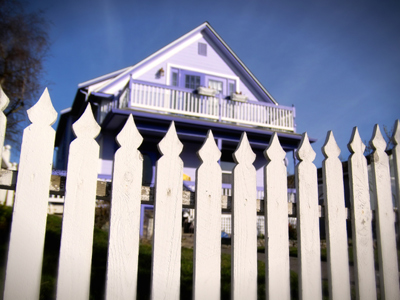It takes Dad 45 minutes to paint 3 fence panels. It takes him 15 minutes to paint one fence panel.

# Solving Problems 2

This Math quiz is called 'Solving Problems 2' and it has been written by teachers to help you if you are studying the subject at elementary school. Playing educational quizzes is an enjoyable way to learn if you are in the 3rd, 4th or 5th grade - aged 8 to 11.

It costs only \$12.50 per month to play this quiz and over 3,500 others that help you with your school work. You can subscribe on the page at Join Us

We come across real problems all the time - How long will it take you to walk to school? How much money will you have left if you buy a packet of candies? - but how do we go about solving them? Solving problems is about finding solutions. This quiz gives you some questions based on everyday scenarios and tests your knowledge of addition, subtraction, multiplication, division, weights, distances, capacities and time.

1.
A baby needs to take 5ml of medicine 3 times a day. How much medicine does the baby need each day?
10ml
15ml
20ml
25ml
5 x 3 = 15 so three lots of 5ml are 15ml
2.
Laura was playing a game. Her scores were 17, 25 and 32. What was her total score for all 3 games?
54
64
74
84
One way to do this is to add the units first (7 + 5 + 2 = 14) and then add the result to the tens (14 + 10 + 20 + 30 = 74)
3.
Ahmed got up at 7:00. It took him 35 minutes to get ready and 20 minutes to walk to school. What time did he arrive in school?
7:30
7:45
7:55
8:05
7:55 can also be written as five to eight
4.
Two shelves measure 75cm and 83cm. What is their total length in meters?
0.158m
1.58m
15.8m
158m
When converting cm to meters remember that there are 100cm in 1 meter
5.
A large glass holds 300ml and a small glass holds 250ml. A jug has 1 liter of juice. First I fill 1 large glass. How many small glasses can be filled with what is left in the jug?
1
2
3
4
First you need to subtract 300ml from 1 liter. As there are 1,000ml in a liter, the answer is 700ml. Divide this by 250ml and the answer is 2 full glasses with 200ml left over
6.
There are 35 red balloons, 22 blue balloons and 7 white balloons. 27 balloons pop. How many balloons are left?
25
32
35
37
You only need to add the units to work this one out. 3 + 2 + 7 = 14 and 14 - 7 = 7. As only one of the answers ends in a 7 we know it must be right
7.
To bake a cake, 250g of flour is required. If a bag of flour is 1kg how much would be left after baking 3 cakes?
250g
500g
600g
750g
3 x 250 = 750
There are 1,000g in a kg so 1,000 - 750 = 250
8.
It takes Dad 45 minutes to paint 3 fence panels. How long does it take to paint one fence panel?
10 minutes
15 minutes
17 minutes
20 minutes
To work this one out you have to divide 45 by 3
9.
Amy drives 13 miles to her grandmother's and then 8 miles to the shops. How many miles has Amy driven?
21
22
23
24
13 + 8 = 21
10.
Mom weighed 55kg. She lost 500g when she was ill. What does she weigh now?
50kg
50.5kg
52.5kg
54.5kg
Remember there are 1,000g in 1kg so 500g is equivalent to half a kg or 0.5kg
Author:  Amanda Swift